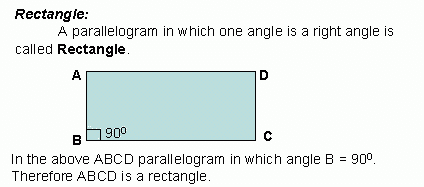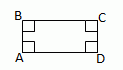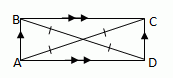Email us to get an instant 20% discount on highly effective K-12 Math & English kwizNET Programs!

#### Online Quiz (WorksheetABCD)

Questions Per Quiz = 2 4 6 8 10

### Geometry3.7 RectangleProperties of Rectangle: Opposite sides of a rectangle are equal or congruent. Opposite angles of a rectangle are equal. All its angles are right angles; or The diagonals of a rectangle bisect each other. Each diagonal divides the rectangle into two congruent triangles. The diagonals of a rectangle are equal. Both pairs of opposite sides are equal and one angle is 90°; or Both pairs of opposite sides are equal and its diagonals are equal. THEOREMS A quadrilateral is a rectangle if and only if it has four right angles.ĐA, ĐB, ĐC, ĐD are 900 A parallelogram is a rectangle, if and only if its diagonals are congruent.AC @ BD Directions: Read the above review points carefully and answer the following questions: Illustrate each of the above review points by drawing a Rectangle. Explain the different properties of a Rectangle, in your own words, with examples. Lilly measures her 10 cm x 6 cm garden plot. She found that all its edges are of angle measure 90°. What will be the shape of her garden? Give reasons. What will be the value of one of the angles of a rectangle if one side is given by 17 cm and other side by 12 cm? Give reasons. If the lengths of the rectangle is x+31 and 5x-9 what is its length. For the following rectangle is the diagonal AC=10, find the mĐDOA, mĐBOC, BO, OD, AO, OC, ACQ 1: "Two congruent triangles can be obtained by drawing a diagonal in a quadrilateral."Falsetrue Q 2: "The diagonals of a rectangle are given by 6 cm and 5 cm."TrueFalse Q 3: Find the measure of angle ODA.Answer: Q 4: In a rectangle, one of the diagonals is 5 cm, then the other diagonal will be _______.10 cmData is not sufficient5 cm Question 5: This question is available to subscribers only! Question 6: This question is available to subscribers only!

#### Subscription to kwizNET Learning System offers the following benefits:

• Unrestricted access to grade appropriate lessons, quizzes, & printable worksheets
• Instant scoring of online quizzes
• Progress tracking and award certificates to keep your student motivated
• Unlimited practice with auto-generated 'WIZ MATH' quizzes